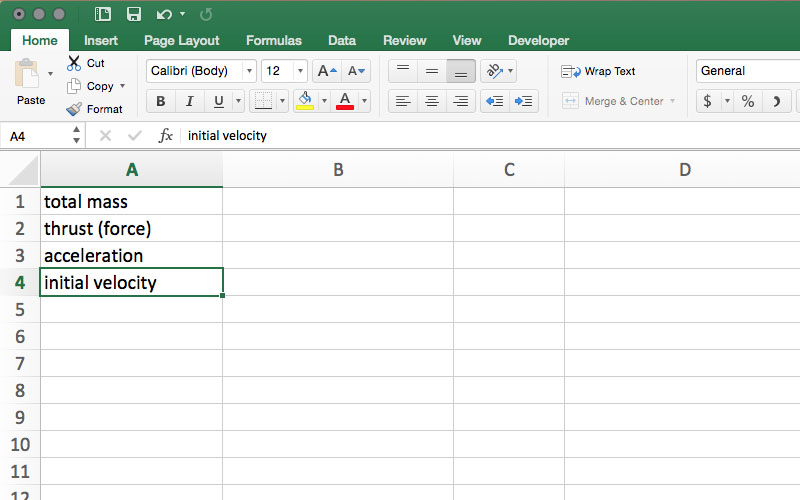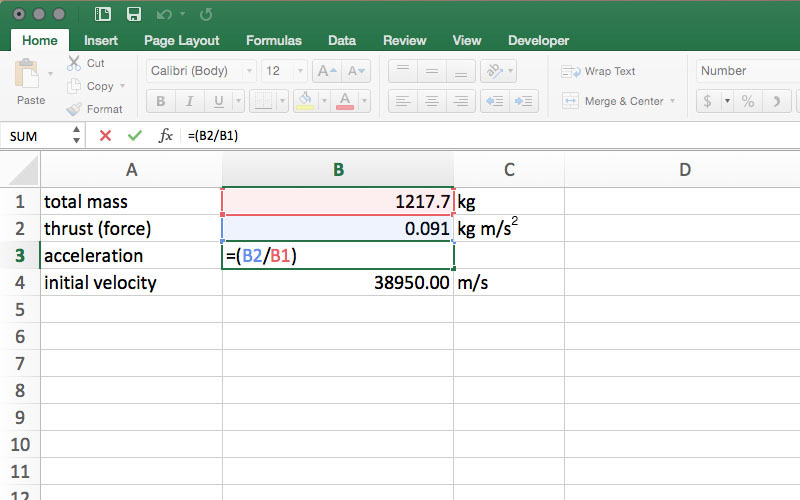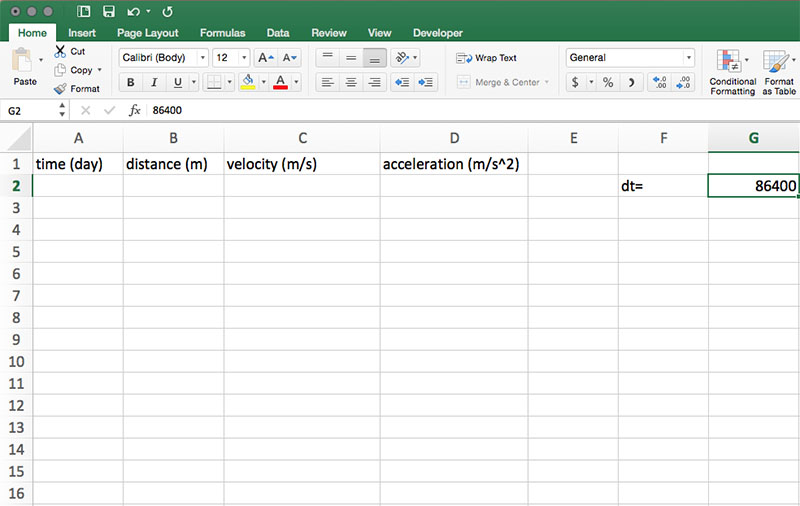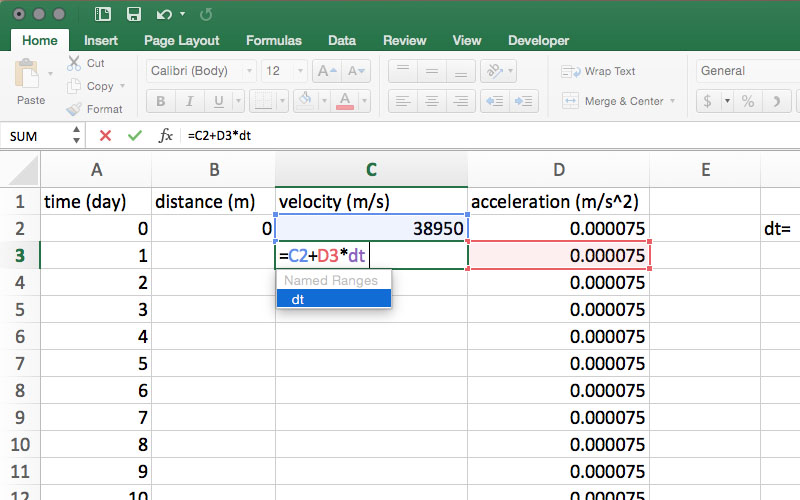This activity is related to a Teachable Moment from May 3, 2016. See "May the Force = mass x acceleration."

› Explore more on the Teachable Moments Blog

### Overview

The Dawn mission and its use of ion propulsion gives students a unique opportunity to study the relationship between mass, force, acceleration, distance and time as described by Newton's Laws of Motion. Students reinforce their knowledge of Newton’s Laws by applying them to a real-world problem: determining the velocity and distance traveled by a hypothetical spacecraft similar to Dawn, and graphing the data they generate.

### Management

• The model will work with a variety of spreadsheet software programs (including Google Sheets), but note the procedure below describes a model created using steps specific to Microsoft Excel.

• This lesson can be used in 1:1 environments, in which each student develops their own model, or in small groups where two to four students work together to develop a model.

• Students less familiar with spreadsheet software may require more support to create the model (e.g., providing some or all the steps needed to create the model). More advanced students may require less support to complete the model. The minimum amount of information that must be provided to the students is included in the Procedures section.

• Students who complete the simplified model can attempt to create a more advanced model described in the Extensions section.

### Background

Launched in 2007, the Dawn Mission was designed to characterize the conditions and processes of the solar system's earliest history by investigating two of the largest protoplanets left intact since they formed. Ceres and Vesta orbit the sun in the asteroid belt between Mars and Jupiter, along with many other smaller bodies. Each formed through a different evolutionary path, influenced by the processes of solar system formation that occurred during the first few million years of our solar system's evolution. By visiting Ceres and Vesta, the Dawn spacecraft allows us to investigate these processes and learn more about the dawn of the solar system.

The Dawn mission's efficient ion propulsion system has allowed Dawn to orbit two different solar system targets. Meeting those ambitious mission objectives would have been impossible without the spacecraft's ion engines. (Read more about how ion propulsion works.)

But more than 300 years before Dawn launched, some of the basic principles that explain how objects orbit the sun and spacecraft travel were first described by Sir Isaac Newton, in his Philosophiæ Naturalis Principia Mathematica. Included in Principia were Newton's Laws of Motion.

Newton's First Law of Motion, also known as the inertia law, states that an object's velocity will remain unchanged unless an outside force acts upon the object. This means that an object that is not moving will remain in place (with a velocity of zero) until an unbalanced force acts on it, causing it to move, while an object in motion will remain at a constant velocity (both speed and direction) until an unbalanced force acts upon it.

The distance (d) traveled by an object traveling at a constant velocity can be calculated by multiplying its velocity (v) by time (t).

d = v · t

Newton's Second Law of Motion describes how the velocity of an object changes when an external force is applied. The law defines a force as equal to change in momentum (mass times velocity) per change in time. For objects with a constant mass, force is equal to the product of mass times acceleration.

F = m · a

When the force units are newtons (N, where 1 N = 1 kg·m/s2), mass (m) is measured in kg and acceleration (a) is measured in m/s2. By rearranging this equation, any variable can be solved for when two other variables are known.

Newton's Third Law of Motion explains that for every action (or force), there is an equal and opposition reaction (force), meaning when a force is applied to an object, that object applies an equal force in the opposite direction.

An understanding of all three of Newton's Laws of Motion helps us to launch spacecraft off of Earth's surface and navigate throughout the solar system.

### Procedures

The following procedures will take you through creating a spreadsheet to calculate the acceleration of a spacecraft, the distance traveled, and the change in velocity over one year after the spacecraft detaches from its launch rocket. You will also create graphs of the data you generate. It is the teacher's discretion how much of this information should be provided to students and how much students will need to figure out on their own.

Students less familiar with spreadsheet software may need to be provided with some (or all) of the steps required to create the model. More advanced students may require less information to complete the model. At a minimum, students must be provided with the following information:

• initial velocity = 38,950 m/s
• total mass = 1217.7 kg
• ion engine thrust = 0.091 N (kg·m/s2)

Note: Familiarize yourself with the following steps. They describe one method for creating a model, though you may do or teach things according to what you prefer. Because versions of Excel on Mac- and Windows-based machines vary, and layouts change from version to version, some steps may need to be completed in a slightly different manner, depending on which version you are using.

1. Open a spreadsheet and rename it "data" by right clicking on the Sheet 1 tab at the bottom of the page and selecting Rename. In Column A, enter total mass into A1, thrust (force) into A2, acceleration into A3, and initial velocity into A4. It is not required that the cells are labeled in that order, but the remaining instructions reference the cells in that order.

2.3. In column B, enter the total mass of 1217.7 in B1. This is the mass in kg of the Dawn spacecraft at launch. Because these units are in kilograms, kg should be entered into C1. In cell B2, enter 0.091. This is the force in newtons (N) applied to the spacecraft by the ion engines. For this spreadsheet, rather than label newtons N, enter kg·m/s2 in cell C2. In cell B4, enter 38,950. This is the spacecraft's highest speed relative to the sun after being released from the rocket that launched it from Earth. This speed is in meters per second, so enter m/s in cell C4.

4.5. By rearranging F = m·a, we see that a = F/m. In cell B3, type the formula =(B2/B1) to get the spreadsheet to calculate acceleration.

6.7. Once =(B2/B1) is entered into cell B3, the spreadsheet will calculate the value for acceleration. In this model, the result is very small, so small, in fact that the default number format in many spreadsheet programs will show zero in cell B3. In order to see the full result, format the cell as a number and increase the number of visible decimal places until 0.000075 is visible in cell B3. Acceleration units are m/s2, so in cell C3, enter m/s2.

8.9. References to cells will be made on a separate sheet within the workbook. To simplify the references, define names for cells B1, B2, B3 and B4 by right clicking on the individual cells and selecting "Define Name..." from the drop-down menu. In the window that appears, a suggested name will appear (e.g., total_mass for cell B1) that can be kept as is or changed to any desired name, but keep in mind that these instructions assume the use of the suggested name. Repeat this process for each cell in column B. In older versions of Excel, click the cell to be named, then select "Name" from the "Insert" menu and click "Define..."

10.11. Add a new sheet by clicking the Insert Sheet plus (+) sign next to the data tab at the bottom of the page. Rename this sheet "calculations."

12. This model calculates and graphs distance, velocity and acceleration in days, but the time units used on the data sheet are seconds. To calculate the distance traveled in one day, we need to multiply velocity by the number of seconds in one day (86,400). So, in cells A1, B1, C1 and D1 enter time (day), distance (m), velocity (m/s), and acceleration (m/s2), respectively. In cell F2, enter dt= and in G2, enter 86,400. Define the name of cell G2 as described in Step 5.

13.14. In cell A2, enter 0. This column represents the number of days that have elapsed since the ion engines began thrusting. The zero indicates that the engine has just started. In cell B2, enter 0. This column represents the distance traveled since the engine began thrusting. In cell C2, enter =initial_velocity. This causes the software to use the value that was entered in the cell named initial velocity. In this case, you should see 38,950 appear. In cell D2, enter =thrust__force/total_mass. This calculates the acceleration of the spacecraft. As was done in Step 4, cell D2 may need to be formatted as a number with enough decimal places to display 0.000075

15.16. In cell A3, enter =A2+1 to indicate one day of elapsed time. With cell A3 selected, click and hold the square that is in the lower right corner of the cell. Drag the corner down over the other cells in Column A to cell A367 to autofill the cells with days for an entire year. Days 1-365 should appear in Column A.

17.18. Select cell D2 and, as described in Step 9, click and drag to autofill cells D3-D367 with the spacecraft acceleration.

19. To calculate the velocity after one day of using the ion thruster, multiply the acceleration in D3 by dt (the number of seconds in a day) and add it to the velocity from the previous day. To do that, in cell C3, enter =C2+(D3·dt)

20.21. In a manner similar to that described in Step 4, select cell C3 and reduce the number of visible decimal places until a whole number appears in the cell. Click and drag from C3-C367 to autofill the velocity formula for the remainder of the year.

22.23. In cell B3, enter =C2·dt to calculate the distance traveled in one day at the velocity in C2.

24.25. In cell B4, enter =B3+(C3·dt) to calculate the distance traveled in the second day, plus the distance traveled in the first day, giving the total distance traveled in two days.

26.27. Select cell B4 and click and drag to autofill cells B5-B367

28. Select all of column C. Click on the "Charts" or "Insert" tab (depending on your version of Excel) and choose "Line" from the available charts. A line graph showing the change in velocity over time should appear. The chart can be edited to include a title and axis labels.

29. To create a graph displaying the total distance traveled, repeat Step 16 using column B as your selected source of data.

30.### Discussion

Models, like the one created in this lesson, are only as accurate as the inputs used to create the model. In this case, there are many factors that influence the speed of the Dawn spacecraft that are not used in these velocity calculations. So for that and other reasons, this model won't match the Dawn spacecraft's velocity at a given time during the mission. Ask students what influences affect the accuracy of this model. Their answers might include:

• The sun's gravity is constantly pulling on the Dawn spacecraft, which continually applies a slowing force, and is a force not accounted for in this model.

• Gravity from Earth, Vesta, Ceres and other bodies in the solar system pull on Dawn, thus changing its velocity.

• Dawn received a boost in its velocity by using what is called a gravity assist when it flew past Mars (click here for an explanation of the gravity assist).

• The model assumes the ion engine is thrusting constantly, when in reality, sometimes the engine is on, and sometimes it is off.

• The ion engines on the Dawn spacecraft must be throttled down when Dawn is too far from the sun to produce enough electrical power for maximum thrust. This model assumes the thruster is being used at full capacity.

• As the ion thruster is powered, fuel is used and the mass of the spacecraft decreases, thereby changing acceleration.

• Thrusters to control the spacecraft's orientation (but not velocity) consume hydrazine fuel, and as they operate, the mass of the spacecraft decreases, changing acceleration.

• The model uses instantaneous velocity and acceleration at 24-hour intervals, rather than every second.

Students can read more about some of the influences acting on the Dawn spacecraft on chief engineer Marc Rayman's Dawn Journal.

### Assessment

1. What is the acceleration experienced by the spacecraft?
0.000075 m/s2, the answer can be found in cell B3 on the data page.

2. What is the total change in velocity in meters per second over the course of one year?
In spreadsheet software, the answer can be found by entering =C367-C2 in any empty cell on the calculations page.

3. How much farther did the spacecraft travel during the last thirty days of the year than in the first thirty days of the year? Why is there a difference?
In spreadsheet software, the answer can be found by entering =(B367-B338)-(B31-B2) in any empty cell on the calculations page.
The spacecraft traveled farther during the last thirty days of the year because the cumulative acceleration results in a higher velocity, thus a great distance traveled.

4. What is the distance in kilometers traveled in the one year since the spacecraft detached from its rocket?
1.26539E+12 meters or 1,265,390,000,000 meters = 1,265,390,000 km. The answer can be found in cell B367 on the calculations page.

5. Is the distance traveled a linear or nonlinear graph?
Nonlinear. Even though the graph may appear to be a straight line, it has a slight curve. This is because each day the spacecraft is traveling faster than the day before, and therefore traveling a greater distance each day. The curve is easier to see when the calculations are carried out to 2,000 days as described in the Extensions section below.

6. What would happen to the rate of acceleration if the force of the ion thrusters were increased? Decreased?
If the force of the ion thrusters were increased, the rate of acceleration would increase. If the force of the ion thrusters were decreased, the rate of acceleration would decrease.

### Extensions

Students can refine the model in the following ways:

1. Extend the model calculations to 2,000 days and adjust the charts to include all the calculations.

2. Adjust the model to show acceleration and velocity if the spacecraft had no hydrazine fuel. (Fully fueled, the spacecraft holds 45.6 kg of hydrazine).

3. Adjust the model to account for the use of xenon at a rate of 3.25 g/s

Excel contains the functionality to create sliders that would allow students to "throttle" the thrust and adjust the amount of fuel in the tanks. Add these requirements in for an added challenge.

Explore More!